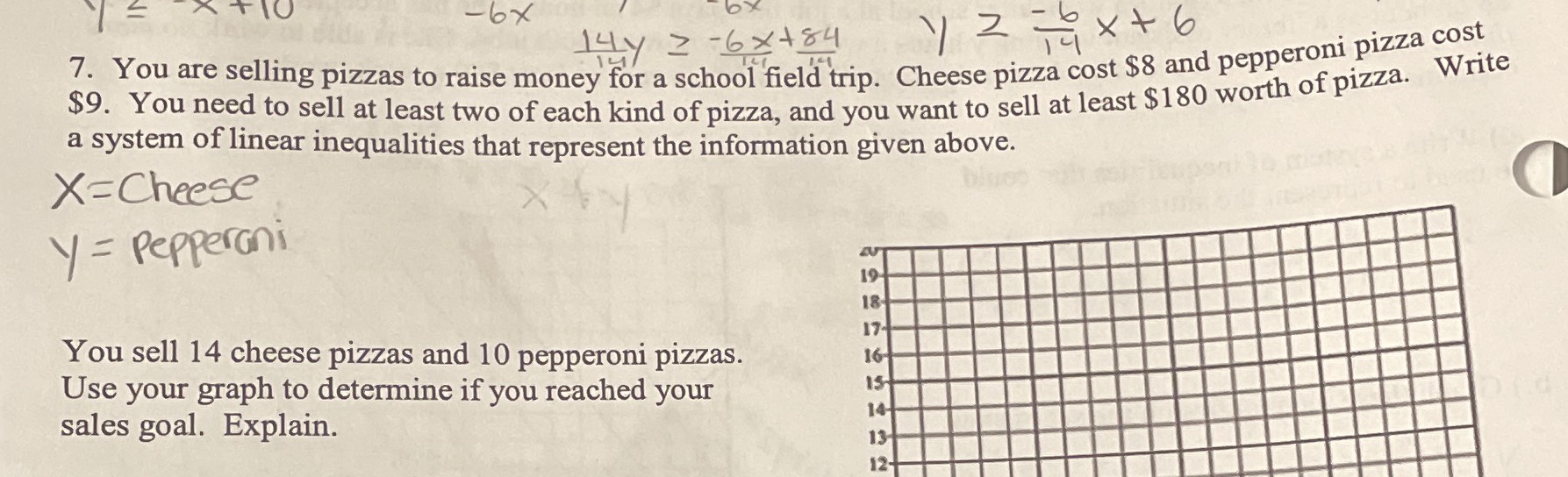### ¿Todavía tienes preguntas de matemáticas?

Pregunte a nuestros tutores expertos
Algebra
Pregunta7. You are selling pizzas to raise money for a school field trip. Cheese pizza cost $$\ 8$$ and pepperoni pizza cost  . You need to sell at least two of each kind of pizza, and you want to sell at least $$\ 180$$ worth of pizza. Write a system of linear inequalities that represent the information given above. You sell $$14$$ cheese pizzas and $$10$$ pepperoni pizzas. Use your graph to determine if you reached your sales goal. Explain.

8x + 9y $$\ge$$ 180

x$$\ge$$2

y$$\ge 2$$

8(14) + 9(10) = 202 $$\ge$$ 180,   yes we reached the goal.

Solución
View full explanation on CameraMath App.